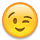When trying to get a good handle on my loads, when the power factor is less than 1.0 should I use the "VA" reading rather than the Watts reading to accurately calculate my loads. For instance a fireplace fan that I use often has a power factor of .49 so my VA reading is about 80 VA but my watts reading is about 40 watts. Thats a big difference when trying to determine my load to accurately size my system. Thanks for any help.

Yes.
.
.
.
.
.
.
.
.
.Basically, you have to use both sets of information to properly size your system.

Watts is equal to V*A x Power Factor when PF=1...

Watts is what we use to size the battery bank and solar array (more or less, you can assume PF=1 for DC power).

V*A deals with the real voltage and "total" current... Because current "lags" voltage when driving AC motors (and is non-linear short current spikes for many electronic power supplies) the wiring and transformers get hot (and other issues) with I^2*R heating (I squared R) effects...

Notice, if because of current lagging (say PF=0.5) or a simple AC battery charger with just diode rectifier front-end--The current needed to operate the load is 2x higher than is really used in "power" (watts)--So the wiring and AC Inverter could overheat if not designed to manage the 2x larger current.

For the smaller stuff we use--Typically the smaller gensets, inverters and such assume Watts=VA rating (i.e., PF=1)...

So, for your load your 40 Watt Load will draw 40 watts (plus losses) from the DC Battery bank, but the AC inverter needs to be rated for 80 Watts--Or, really 80 VA.

Some larger gensets I have seen are rated differently between watts and VA--For example you may see a 10 kVA rated genset with a 6kVW output (assuming PF=0.6 -- typical for driving electric motors).

So, if you have a 1,200 watt inverter that runs on 12 VDC... The maximum continuous DC current would be:
• 1,200 Watts * 1/10.5 volts min DC voltage * 1/0.85 inverter eff * 1.25 NEC safety factor = 168 amp minimum circuit...
The same inverter driving a 200 VA motor with 0.6 PF would typically see:
• 200 VA = Inverter Rating (in VA)
• 200 VA * 0.6 pf = 120 watts inverter load
• 200 VA / 120 Volts = 1.67 amps @ 120 VAC
• 120 watts * 1/12 volt battery * 1/0.85 inverter eff = 11.8 amps @ 12 VDC from battery
The same 200 VA load supporting an electric heater (or desktop computer with power factor corrected supply) would see:
• 200 VA = Inverter Rating (in VA)
• 200 VA * 1.0 PF = 200 Watts inverter rating
• 200 VA / 120 VAC = 1.67 Amps @ 120 VAC
• 200 Watts * 1/12 volt battery * 1/0.85 inverter eff = 19.6 amps @ 12 volts from battery
So, bad Power Factors require a larger inverter to support the V*A of the loads.

But good or bad Power Factor does not matter to your battery bank or solar array (within reason, a bit more losses associated with bad power factors).

-Bill
Near San Francisco California: 3.5kWatt Grid Tied Solar power system+small backup genset

With power factors being so important, where do you get a PF rating for a product? Say a desktop computer?
bobdog wrote: »
With power factors being so important, where do you get a PF rating for a product? Say a desktop computer?

A Kill-A-Watt will actually give you the real numbers. http://www.solar-electric.com/kiacpomome.html

Now that explains why the kill-a-watt meter is different than the rating on the particular item. Thanks. That cleared that up as well.
• Solar Expert Posts: 1,394 ✭✭✭✭

Maybe.

Depends on what you are doing.

If for grid tied power on residential use, you pay for true power (watts). Power factor means little more then very slightly higher losses in residential wiring.

Industry heavy users get charged a penalty for poor power factor.

For an off grid inverter, it depends a bit on type of inverter, but generally your batteries provide true power (watts) plus any additional inverter losses equivalent to inverter running a wattage load equivalent to VA number.

For example, a 4000 watt inverter that yields a 90% efficiency for a 4000 watt resistive load has 444 watts loss in inverter (heat) and consumes 4444 watts from batteries.

A 4000 VA load with a PF of 0.5 will consume 2000 watts + 444 watts = 2444 watts from batteries, for an 82% equivalent inverter efficiency. The 2000 watts is the true power component of the load and the 444 watts is the inverter loss which is equivalent to inverter running a 4000 watt load.

Generators and Inverters are rated at VA since their I^2*R losses are what matters to them.

Inexpensive unidirectional inverters (like Power-to-Go) cannot support a rated VA load with a PF worse then about 0.6 to 0.7. Since the unidirectional inverter cannot push reactive power back to batteries they can blow out with high VA of poor PF.